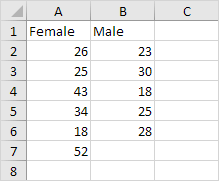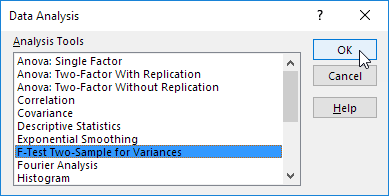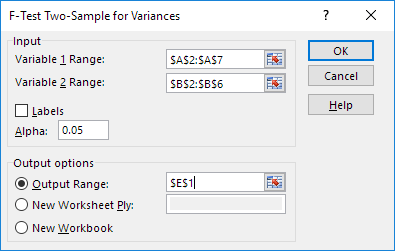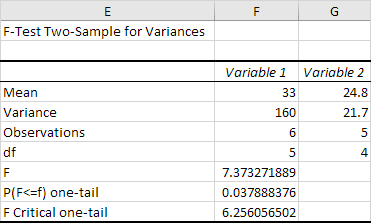# F-Test

This example teaches you how to perform an F-Test in Excel. The F-Test is used to test the null hypothesis that the variances of two populations are equal.

Below you can find the study hours of 6 female students and 5 male students.

H0: σ12 = σ22
H1: σ12 ≠ σ22To perform an F-Test, execute the following steps.

1. On the Data tab, in the Analysis group, click Data Analysis.2. Select F-Test Two-Sample for Variances and click OK.3. Click in the Variable 1 Range box and select the range A2:A7.

4. Click in the Variable 2 Range box and select the range B2:B6.

5. Click in the Output Range box and select cell E1.6. Click OK.

Result:Important: be sure that the variance of Variable 1 is higher than the variance of Variable 2. This is the case, 160 > 21.7. If not, swap your data. As a result, Excel calculates the correct F value, which is the ratio of Variance 1 to Variance 2 (F = 160 / 21.7 = 7.373).

Conclusion: if F > F Critical one-tail, we reject the null hypothesis. This is the case, 7.373 > 6.256. Therefore, we reject the null hypothesis. The variances of the two populations are unequal.

Go to Next Chapter: Create a Macro